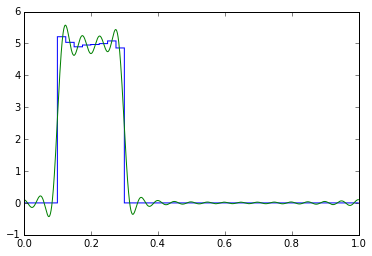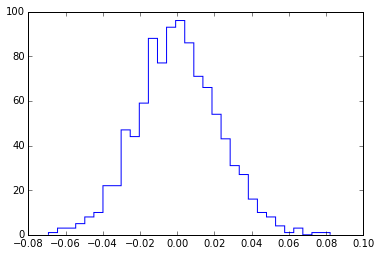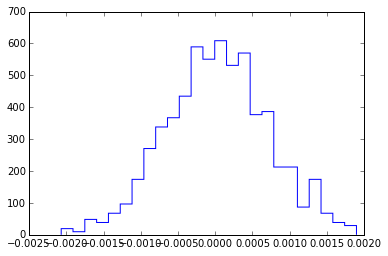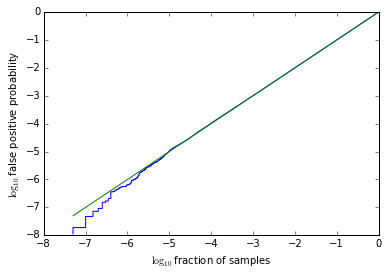### Parallel ipython notebooks and the H test

The CPU on the nice shiny new server I log in to is really not much faster than that of the ratty old laptop I have in front of me. The server has more memory, and more disk space, but CPU-wise it just has more not faster. For that matter even my laptop has two cores. So if I have some heavy-duty computing task, I'd better find a way to make it use multiple cores in parallel. Some tasks are just plain hard to parallelize (solving ordinary differential equations, for example), but it turns up fairly often that I'm doing something embarrassingly parallel: there's a for loop somewhere that just does the same thing to lots of different pieces of input. If only it were easy to hand each piece to a different core! Well, there are various tools for doing this sort of thing, but most of them apply to scripts or programs that run non-interactively. It turns out that ipython offers tools for interactive parallel computing. I'm going to explain how I use them, by working through a test problem, checking some statistics on a periodicity test (the H test).

I should say first that getting ipython parallelization working falls into two parts: one is writing scripts that use the parallel facilities, and the other is getting the parallel facilities using all the cores you have available (on your cluster, say). Fortunately if you don't do much of anything to set them up, you can easily just run multiple processes on the machine your notebook is running on, so I'll talk about the scripts first and then go into how you'd get them to use a batch system.

The general structure of an ipython parallel notebook involves a "controller" and a set of "engine" processes. The controller process allocates jobs to engines but doesn't do any computation itself; the parallel facilities let you ship data and commands out to the engines for computation. One thing to watch out for is that it's easy to accidentally end up with more than one ipython process talking to the same controller and group of engines; since each engine has its own singular python interpreter, having multiple notebooks defining variables there can lead to, um, confusion.

If you use a modern ipython notebook server, the server page will have a tab listing notebooks and a tab listing "clusters", by which they mean groups of engines, one per profile. There should be one for "default"; you can start a bunch of engines by typing the number in there and hitting "start". I'm going to use the profile "slownodes", which I've set up to use the cluster I'm working on. Note that it may take a few seconds for all the engines to wake up and report in.

In :
from IPython import parallel
clients = parallel.Client(profile="slownodes") # lust leave out the profile argument to get the default
print clients.ids

[0, 1, 2, 3, 4, 5, 6, 7, 8, 9, 10, 11, 12, 13, 14, 15, 16, 17, 18, 19, 20, 21, 22, 23, 24, 25, 26, 27, 28, 29, 30, 31, 32, 33, 34, 35, 36, 37, 38, 39]



Good, there is a decent collection of engines (that list of ids) up and running. ipython offers two different objects for submitting jobs to engines. A "direct view" simply submits the same objects to all of them, while a "load balanced view" lets them grab a new job as soon as they finish. We're going to want both.

In :
view = clients.load_balanced_view()
dview = clients.direct_view()


A quick test, to see which machines we're actually running on. The "dview.remote" decorator just makes the function it's annotating run on all the engines instead of the controller. Nimrod was "a mighty hunter before the Lord", to quote the cluster's motd.

In :
import platform
platform.node()

Out:
'nimrod'

In :
@dview.remote(block=True)
def nodename():
import platform
return platform.node()

r = nodename()
print len(r), len(clients.ids)
r

40 40


Out:
['nimrod04',
'nimrod04',
'nimrod06',
'nimrod04',
'nimrod04',
'nimrod04',
'nimrod04',
'nimrod04',
'nimrod06',
'nimrod05',
'nimrod05',
'nimrod05',
'nimrod05',
'nimrod06',
'nimrod06',
'nimrod04',
'nimrod07',
'nimrod07',
'nimrod07',
'nimrod07',
'nimrod05',
'nimrod07',
'nimrod07',
'nimrod07',
'nimrod07',
'nimrod06',
'nimrod05',
'nimrod05',
'nimrod06',
'nimrod06',
'nimrod05',
'nimrod06',
'nimrod08',
'nimrod08',
'nimrod08',
'nimrod08',
'nimrod08',
'nimrod08',
'nimrod08',
'nimrod08']


The engines are separate python interpreters, so I need to make sure things are imported there appropriately. For real imports, the sync_imports arranges imports to happen both on the controller and all the engines. For things like the future imports that are really syntax switches, I have to run it by hand on the controller and then use an ipython magic to run it on all the nodes. Also sync_imports doesn't understand import as, so I have to do that by hand too.

In :
from __future__ import division
%px from __future__ import division
with dview.sync_imports():
import numpy
import scipy.stats
import scipy

In :
%px import numpy as np


All right, the machines seem to be working, now for the math.

## The H test

The H test is a test for periodicity in events. Say you have some photons from an X-ray pulsar, and you have worked out the arrival phase of each one. Are these phases distributed uniformly on $(0,1)$, or are they clustered around certain phases, indicating that the X-rays are at least partially modulated at the period you used to get the phases?

It's not usually done this way, but I prefer to think of the H test in two steps. The first step is to build the "empirical Fourier coefficients", and the second is to look whether those coefficients look like noise or whether they show some evidence of periodicity.

The empirical Fourier coefficients for a collection of phases $p_j$ are $c_k = \frac{1}{n}\sum_{j=1}^{n} e^{-2\pi i k p_j}.$ If the phases come from some distribution, then the $c_k$ will gradually converge to the Fourier coefficients of that distribution.

In :
p = np.random.uniform(0.1,0.3,size=10000)
c = np.zeros(20,dtype=np.complex)
for k in range(len(c)):
c[k] = np.sum(np.exp(-2.j*np.pi*k*p))/len(p)
plt.hist(p,bins=2*len(c), range=(0,1),histtype='step',normed='true')
xs = np.linspace(0,1,1024,endpoint=False)
plt.plot(xs,np.fft.irfft(c,n=len(xs))*len(xs))

Out:
[<matplotlib.lines.Line2D at 0x32624810>]The H test looks at whether these coefficients look like noise. (Obviously the ones I just generated shouldn't.) So what is the distribution if we really feed in noise? Well, noise means random phases, so each Fourier coefficient looks like the result of a random walk with $n$ steps of size $1/n$. So in this case the real and imaginary parts should be (approximately) normally distributed with mean zero and standard deviation $1/\sqrt{2n}$.

Let's test this by generating some random sets of photons, computing a Fourier coefficient, and checking its distribution.

In :
n = 1000
def gen_coefficient():
p = np.random.uniform(size=n)
c = np.sum(np.exp(-2.j*np.pi*p))/len(p)
return c.real

In :
cs = [gen_coefficient() for i in range(1000)]

In :
print "std dev:", np.std(cs)
print "std dev over formula:", np.std(cs)/(1/np.sqrt(2*n))
plt.hist(cs,
bins=np.sqrt(len(cs)),
histtype='step')
scipy.stats.anderson(cs)

std dev: 0.0220106915027
std dev over formula: 0.984348048636


Out:
(0.24433129807880505,
array([ 0.574,  0.653,  0.784,  0.914,  1.088]),
array([ 15. ,  10. ,   5. ,   2.5,   1. ]))That scipy.stats.anderson is a test for deviations from normality; the first value it returns is the test statistic, the second is an array of thresholds, and the third is the percentile significances of the thresholds. Short answer is, this looks like a normal distribution. Let's try it with more photons (which should be fine, but I want a job that's computationally expensive).

Now we get to the parallel part: we're going to need to generate and sum up an awful lot of photons (more than the million we just used), so let's let the engines do that. So here I'm going to use an idiom I've dound useful in the past that lets me easily look at partial results and accumulate more without restarting. But first I have to make sure all the various things I want to use on the engines are available.

This first block is the setup, initializing the results to empty and clearing (but not killing! I don't think you can) any queued jobs.

In :
n = 1000000

In :
dview.push(dict(n=n),block=True)
async = []
results = []


This block queues up another thousand jobs. Run it as many times as needed; they go into the queue.

In :
async.extend([ view.apply(gen_coefficient)
for i in range(1000)])


This block pulls all finished jobs off the queue and summarizes the progress so far. You can go back and add more jobs if necessary. If you have a big cluster it may not be easy to catch the jobs not all finished; come up with something more computational and you'd have no problem.

In :
new_async = []
for a in async:
results.append(a.result)
else:
new_async.append(a)
async = new_async
print len(results), "done"
print len(async), "still in progress"

652 done
348 still in progress


In :
cs = np.array(results)
print "std dev:", np.std(cs)
print "std dev over formula:", np.std(cs)/(1/np.sqrt(2*n))
plt.hist(cs,
bins=np.sqrt(len(cs)),
normed=True,
histtype='step')
scipy.stats.anderson(cs)

std dev: 0.000688445156686
std dev over formula: 0.973608477536


Out:
(0.11956254832853119,
array([ 0.573,  0.652,  0.782,  0.912,  1.085]),
array([ 15. ,  10. ,   5. ,   2.5,   1. ]))Sorry, I realize that test wasn't really worth the heavy-duty computing. We know the distribution of a random walk. I'm just going to put some math in the next batch and I didn't want the math and the parallelization to appear at the same time.

Now for the second half of the H test: checking whether the Fourier coefficients look like noise. The key idea of the H test is that there is an optimal number of harmonics to use to represent the profile. Specifically, if you choose the number of harmonics $m$ to maximize $S_m = \sum_{k=1}^m |\sqrt{2n}c_k|^2 - 4,$ then you are also minimizing an estimator of the mean integrated squared error between the Fourier profile and the true profile. (This is called Hart's rule, and it works because the mean integrated squared error has two components, one due to the noise in the measured coefficients, and another due to the truncation of the true profile's Fourier series; this choice balances the two in a well-defined way.) In other words, buried in the H test is a way to find an optimal Fourier representation of the profile - analogous to a data-driven rule for how many bins to use in a histogram. (This is related to the theory of kernel density estimators in statistics, though on the circle.)

The H test looks for the presence of a signal in the noise; it uses the value of $S_m$ for the optimal $m$ as its statistic. Usually it only looks at the first $20$ harmonics, but the choice of $20$ is somewhat arbitrary; by the time you get a statistically-significant $20$th harmonic, your profile is normally so bright there's no question of detection any more, so there's not much to be gained by considering higher harmonics. The distribution of this number, the best $S_m$, was worked out in Kerr 2011, so that you can write the false positive probability (the chance that noise would produce a distribution with this much Fourier power) as $\log FPP = -0.398405 \max_{m=1}^{20} \sum_{k=1}^m |\sqrt{2n}c_k|^2 - 4.$

In :
def H(norm_c):
return -0.398405*np.amax(np.cumsum(np.abs(norm_c[1:])**2-4)+4)
H(c*np.sqrt(2*len(p)))/np.log(10)

Out:
-6662.9607958139168


So that profile from earlier is clearly detected (as it should be). But what matters is whether the claimed false positive probability is really right; to do that I'm going to want to feed in a lot of noise and see how often different values come out. And because I want to test it out to quite small false positive probabilities, I'm going to want to run a lot of simulations. Parallelization ahoy!

I'm going to use the same trick as above, only I happen to know that one simulation is fast and shipping jobs back and forth is slow, so I'm going to send them out in batches.

In :
def run_batch():
r = []
for i in range(1000):
norm_c = (np.random.randn(21)
+1.j*np.random.randn(21))
r.append(H(norm_c))
return r

In :
dview.push(dict(H=H),block=True)
async = []
results = []

In :
async.extend([ view.apply(run_batch)
for i in range(1000)])

In :
new_async = []
for a in async:
results.extend(a.result)
else:
new_async.append(a)
async = new_async
print len(results), "done"
print len(async), "batches still in progress"

20000000 done
0 batches still in progress



If these are really false positive probabilities, then a fraction $f$ will be less than or equal to $f$, for all $f$. So we can plot just that.

In :
r = results[:]
r.sort()
r = np.array(r)
plt.plot(np.log10((np.arange(len(r))+1)/len(r)),
r/np.log(10), drawstyle="steps")
plt.plot([-np.log10(len(r)),0],[-np.log10(len(r)),0])
plt.xlabel(r"$\log_{10}$ fraction of samples")
plt.ylabel(r"$\log_{10}$ false positive probability")

Out:
<matplotlib.text.Text at 0x3276aed0>So it looks like this is good out to at least one in a million; the math says it should be better than that, but this test also serves as a good check on my implementation (which I got wrong on the first try).

## Setting up ipython to run its engines on a cluster

You need a profile to keep track of your ipython settings. So make one with ipython profile create --parallel profilename. This should make a directory ~/.config/ipython/profile_profilename/ where you will want to edit the files. The exact details of what needs to go there will depend on your cluster, and I haven't done this for enough clusters to be sure I'm explaining in full generality. But the documentation is a little sparse, so I'm going to write this out and wish you luck.

The cluster I'm working on uses PBS for job control. So ipython has to submit a job to PBS to get its engines - and optionally another for its controller - run. First we tell ipython (actually the tool ipcluster) that: in ipcluster_config.py set c.IPClusterStart.controller_launcher_class = 'PBS' and c.IPClusterEngines.engine_launcher_class = 'PBS'.

Next we have to give ipcluster scripts to start the controller and the engines; these will be fed to qsub, and they contain all the various details PBS needs. They can go in external files but I prefer to embed them directly in ipcluster_config.py as triple-quoted strings. So set c.PBSControllerLauncher.batch_template to:

#!/bin/sh
#PBS -lnodes=nimrod:ppn=1
#PBS -N ipython_controller
#PBS -V

cd $PBS_O_WORKDIR export PATH=$PBS_O_PATH
ipcontroller --profile-dir={profile_dir}

The arcane thing here is the PBS options. -N simply sets the job name, and -V makes sure things like PYTHONPATH (but not PATH; hence the export) get passed in. The -l specifies how many nodes, processors per node, and what kind to use. This is a little tricky; because this is the controller, which doesn't really do any work, I actually want it to run on the cluster head node instead of occupying a real worker node. So I specify the head node by name. Of course this means that if the head node gets full (whether or not it's actually busy) I'm in trouble. But it's the engines I'm trying to allocate efficiently. For that we'd set c.PBSEngineSetLauncher.batch_template to:
#!/bin/sh
#PBS -lnodes={n//8}:ppn=8:compute:old
#PBS -N ipython_cluster
#PBS -V

cd $PBS_O_WORKDIR export PATH=$PBS_O_PATH
mpiexec -n {n} ipengine --profile-dir={profile_dir}

This took some sweating. Since these jobs don't communicate much with each other, I would prefer to simply allocate nodes={n}:ppn=1, that is, one node per job and one processor per node. Normally, PBS will schedule jobs on the same nodes as long as there are processors left, so this would mean the jobs get stuck wherever, with up to 8 per node as needed. Unfortunately that's not what happens on this cluster, for some reason; nodes={n} really means n *different* nodes for this job, even though PBS will put other jobs on the same nodes. So I have to work in groups of 8, using all of a node at a time. (If I schedule a non-multiple of 8 jobs, MPI just sticks extra processes on some of the nodes.) Anyway, the :compute:old are node flags (discoverable with pbsnodes) that make sure this job actually goes on the nodes I want. (The new nodes are running a monster MCMC fit for me just now.)

Finally, all the engines need to be able to look up the controller. Since they're on different machines, the controller needs to listen on a network-accessible port. Ignoring security considerations, you can usually just tell it to listen on all interfaces on the controller machine, but in my case the head node is visible to the outside world, but the compute nodes can't reach it using its public IP address, so I need to look up the private IP address of the head node with ifconfig and put it in ipcontroller_config.py: c.HubFactory.ip = u'10.23.23.23' (that's not the real IP address).